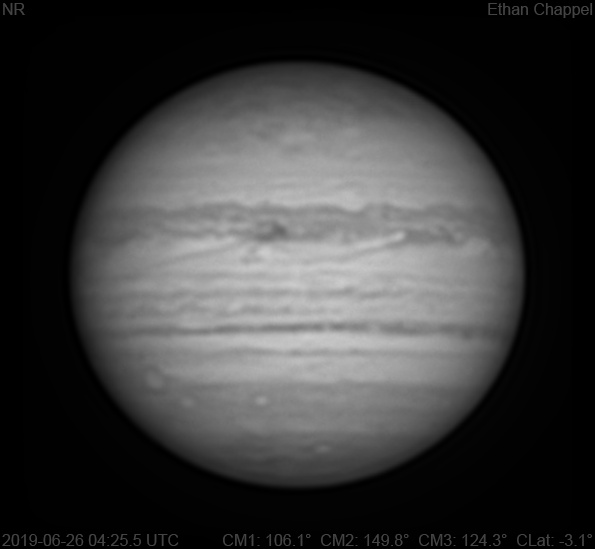# Jupiter 2019-06-26 04:25 UTC

CM1: 106.10°

CM2: 149.80°

CM3: 124.30°

CLat: -3.10°

Description

The sky continued to defy an unfavorable forecast, so I hastily captured a near infrared image before it ended.

Notes:

• A long rift on the North Equatorial Belt is approaching the central meridian.
• The southern South Equatorial Belt is very dark.
• Oval BA is rising.
• The faint streak in the South Temperate I noted on an image from June 2 is at the central meridian.
• The South Polar Red Spot is just east of the CM.

Equipment

ZWO ASI290MM

Celestron EdgeHD 8

Logs
```FireCapture v2.6  Settings
------------------------------------
Observer=Ethan Chappel
Camera=ZWO ASI290MM
Filter=IR
Profile=Jupiter
Diameter=45.65"
Magnitude=-2.59
CMI=106.8° CMII=150.5° CMIII=124.9°  (during mid of capture)
FocalLength=3750mm (F/13)
Resolution=0.16"
Filename=2019-06-26-0426_7-EC-IR-Jup.ser
Date=2019_06_26
Start=04_25_42.691
Mid=04_26_42.691
End=04_27_42.691
Start(UT)=04_25_42.691
Mid(UT)=04_26_42.691
End(UT)=04_27_42.691
Duration=120.000s
Date_format=yyyy_MM_dd
Time_format=HH_mm_ss
LT=UT -6h
Frames captured=7997
File type=SER
Binning=no
Bit depth=8bit
Debayer=no
ROI=460x376
ROI(Offset)=0x0
FPS (avg.)=66
Shutter=15.00ms
Gain=280 (46%)
HighSpeed=off
SoftwareGain=10 (off)
AutoExposure=off
AutoGain=off
AutoHisto=75 (off)
USBTraffic=40 (off)
Gamma=50
FPS=100 (off)
Brightness=1 (off)
Histogramm(min)=0
Histogramm(max)=118
Histogramm=46%
Noise(avg.deviation)=0.59
AutoAlign=false
PreFilter=none
Limit=120 Seconds
Sensor temperature=32.1°C
FireCapture v2.6  Settings
------------------------------------
Observer=Ethan Chappel
Camera=ZWO ASI290MM
Filter=IR
Profile=Jupiter
Diameter=45.65"
Magnitude=-2.59
CMI=105.4° CMII=149.1° CMIII=123.5°  (during mid of capture)
FocalLength=3750mm (F/13)
Resolution=0.16"
Filename=2019-06-26-0424_3-EC-IR-Jup.ser
Date=2019_06_26
Start=04_23_21.871
Mid=04_24_21.878
End=04_25_21.885
Start(UT)=04_23_21.871
Mid(UT)=04_24_21.878
End(UT)=04_25_21.885
Duration=120.014s
Date_format=yyyy_MM_dd
Time_format=HH_mm_ss
LT=UT -6h
Frames captured=7998
File type=SER
Binning=no
Bit depth=8bit
Debayer=no
ROI=1248x348
ROI(Offset)=0x0
FPS (avg.)=66
Shutter=15.00ms
Gain=280 (46%)
HighSpeed=off
SoftwareGain=10 (off)
AutoExposure=off
AutoGain=off
AutoHisto=75 (off)
USBTraffic=40 (off)
Gamma=50
FPS=100 (off)
Brightness=1 (off)
Histogramm(min)=0
Histogramm(max)=111
Histogramm=43%
Noise(avg.deviation)=0.60
AutoAlign=false
PreFilter=none
Limit=120 Seconds
Sensor temperature=32.1°C
```